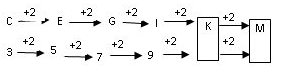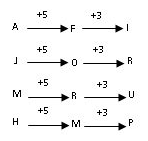Courses

# Amazon Aptitude Paper 2

## 10 Questions MCQ Test Placement Papers - Technical & HR Questions | Amazon Aptitude Paper 2

Description
This mock test of Amazon Aptitude Paper 2 for Quant helps you for every Quant entrance exam. This contains 10 Multiple Choice Questions for Quant Amazon Aptitude Paper 2 (mcq) to study with solutions a complete question bank. The solved questions answers in this Amazon Aptitude Paper 2 quiz give you a good mix of easy questions and tough questions. Quant students definitely take this Amazon Aptitude Paper 2 exercise for a better result in the exam. You can find other Amazon Aptitude Paper 2 extra questions, long questions & short questions for Quant on EduRev as well by searching above.
QUESTION: 1

### DIRECTIONS for the question 1 to 3:  Q. Go through all the options and mark the one which does not belong to the group.

Solution:

248-231 = 17

563-547=16

71-55=16

523-517=06

Except in the number pair 248-231, in all others number pair, the difference between the two numbers is an even number.

QUESTION: 2

### Go through all the options and mark the one which does not belong to the group. Q. 10, 13, 234, 681, 997

Solution:

10 1+0 =1 or 1^2

13 1+3 = 4 or 2^2

234 2+3+4 = 9 or 3^2

681  6+8+1 = 

997 9+9+7 = 25 or 5^2

So the sum of the digits in all the numbers is a perfect square except for 681, hence that is odd.

QUESTION: 3

### Go through all the options and mark the one which does not belong to the group. Q. Choose amongst the Options below.

Solution:

Except in the word Led, in all other words there are two vowels.

Hence the answer is option 2.

QUESTION: 4

DIRECTIONS for the question 4-8:

What should come in place of question mark (?) in the following number/alphabetic series?

Q. C 3, E 5, G 7, I  9, ?, ?

Solution:QUESTION: 5

What should come in place of question mark (?) in the following number/alphabetic series?

Q. 5, 16, 51, 158, ?

Solution:

5 - 3 + 1 = 16

16 - 3 + 3 = 51

51 - 3 + 5 = 158

158 - 3 + 7 = 

QUESTION: 6

What should come in place of question mark (?) in the following number/alphabetic series?

Q. 9 - 2: 9 - 9 : : 9 - 5 : ?

Solution:

9-2=18

9-9=81

The digits of resultant have interchanged positions therefore,

9-5=45

9-6=54

QUESTION: 7

What should come in place of question mark (?) in the following number/alphabetic series?

Q. AFI, JOR, MRU, ?

Solution:QUESTION: 8

What should come in place of question mark (?) in the following number/alphabetic series?

Q. bc, cde, de, efg, fg, ?

Solution:

The answer should be the last alphabhet of fg ( i.e g ) and the next two alphabets i.e hi . Hence answer is ghi

bc cde  de efg  fg  ghi

QUESTION: 9

A man showed a boy next to him and said – “He is the son of my wifes sister-in-law, but I am the only child of my parents.” How is man's son related to him?

Solution:

The boy is the son of man's brother-in-law. Therefore, man's son is the cousin of that boy.

QUESTION: 10

Find the correct group of signs to solve the equation. 24 * 16 * 8 * 32

Solution:

The best way to solve this question is by hit and trial . Use the answer options and find the value.

24 * 16 * 8 * 32

24 + 16 - 8 = 32

40-8 = 32

In this case, option C is correct.